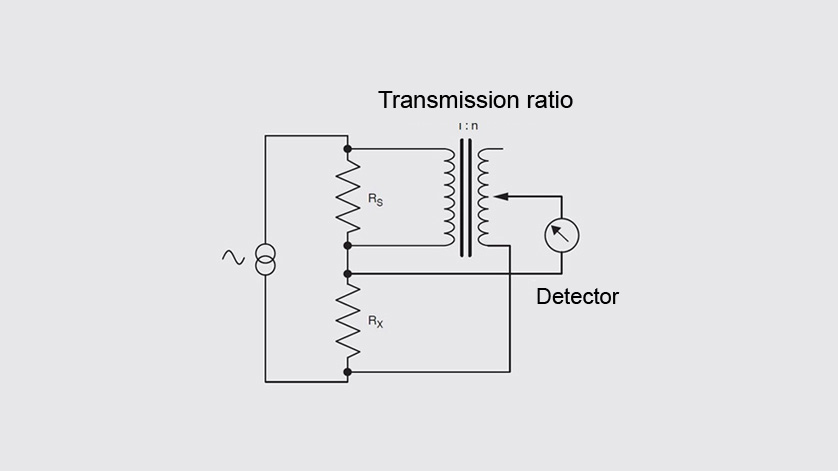When a constant current is passed through a thermometer of resistance Rt and a fixed reference resistor of known value Rs, the voltage across them will be in direct proportion to their resistance values.

The ratio of the two voltages and therefore of the two resistors, can be measured very accurately using high-precision voltage divider techniques employed in the AC bridges with ASL technology. As Rs is known, Rt can be determined from n=Rt/Rs, where n is the measured ratio.

## The advantages of the AC bridge

The low-frequency AC (alternating current) bridge technology has major advantages over DC (direct current) systems for high-precision measurement of platinum resistance thermometers, two of which are:

• DC generates small voltages in the thermometer, reference resistor and cables, across every junction where different materials are used, (for example copper, tin, platinum, palladium, nickel etc.). These voltages add to or subtract from the measured voltages and are dependent on the various temperature differences at the junctions, hence they are referred to as ‘Thermal EMFs’. These variable voltages cause measurement errors and the more accurate DC bridge systems switch the polarity of the current to try to solve the problem, taking between two and four seconds for each reversal. By using the ASL technology, the AC bridges perform this reversal automatically 75 times a second, a much more effective solution.
• Active circuits, which are fundamental to the performance of DC systems, suffer from ambient temperature changes and also the effects of component ageing. Fundamental to the ASL AC bridges accuracy is its inductive voltage divider – a passive, precision voltage divider, the performance of which is unaffected by ambient temperature change and by time. DC bridges require very stable and accurate electronics to achieve their performance. Because active circuitry within the AC bridge is secondary to performance, the effects of active component drifts and ageing are therefore minimised. This results in an instrument which does not require regular recalibration to remain within specification.

Note
Information on our resistance thermometry bridges can be found on the WIKA Website.